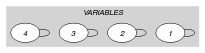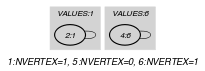## 5.165. global_cardinality_low_up_no_loop

Origin
Constraint

$\mathrm{𝚐𝚕𝚘𝚋𝚊𝚕}_\mathrm{𝚌𝚊𝚛𝚍𝚒𝚗𝚊𝚕𝚒𝚝𝚢}_\mathrm{𝚕𝚘𝚠}_\mathrm{𝚞𝚙}_\mathrm{𝚗𝚘}_\mathrm{𝚕𝚘𝚘𝚙}\left(\begin{array}{c}\mathrm{𝙼𝙸𝙽𝙻𝙾𝙾𝙿},\hfill \\ \mathrm{𝙼𝙰𝚇𝙻𝙾𝙾𝙿},\hfill \\ \mathrm{𝚅𝙰𝚁𝙸𝙰𝙱𝙻𝙴𝚂},\hfill \\ \mathrm{𝚅𝙰𝙻𝚄𝙴𝚂}\hfill \end{array}\right)$

Synonym

$\mathrm{𝚐𝚌𝚌}_\mathrm{𝚕𝚘𝚠}_\mathrm{𝚞𝚙}_\mathrm{𝚗𝚘}_\mathrm{𝚕𝚘𝚘𝚙}$.

Arguments
 $\mathrm{𝙼𝙸𝙽𝙻𝙾𝙾𝙿}$ $\mathrm{𝚒𝚗𝚝}$ $\mathrm{𝙼𝙰𝚇𝙻𝙾𝙾𝙿}$ $\mathrm{𝚒𝚗𝚝}$ $\mathrm{𝚅𝙰𝚁𝙸𝙰𝙱𝙻𝙴𝚂}$ $\mathrm{𝚌𝚘𝚕𝚕𝚎𝚌𝚝𝚒𝚘𝚗}\left(\mathrm{𝚟𝚊𝚛}-\mathrm{𝚍𝚟𝚊𝚛}\right)$ $\mathrm{𝚅𝙰𝙻𝚄𝙴𝚂}$ $\mathrm{𝚌𝚘𝚕𝚕𝚎𝚌𝚝𝚒𝚘𝚗}\left(\mathrm{𝚟𝚊𝚕}-\mathrm{𝚒𝚗𝚝},\mathrm{𝚘𝚖𝚒𝚗}-\mathrm{𝚒𝚗𝚝},\mathrm{𝚘𝚖𝚊𝚡}-\mathrm{𝚒𝚗𝚝}\right)$
Restrictions
 $\mathrm{𝙼𝙸𝙽𝙻𝙾𝙾𝙿}\ge 0$ $\mathrm{𝙼𝙸𝙽𝙻𝙾𝙾𝙿}\le \mathrm{𝙼𝙰𝚇𝙻𝙾𝙾𝙿}$ $\mathrm{𝙼𝙰𝚇𝙻𝙾𝙾𝙿}\le |\mathrm{𝚅𝙰𝚁𝙸𝙰𝙱𝙻𝙴𝚂}|$ $\mathrm{𝚛𝚎𝚚𝚞𝚒𝚛𝚎𝚍}$$\left(\mathrm{𝚅𝙰𝚁𝙸𝙰𝙱𝙻𝙴𝚂},\mathrm{𝚟𝚊𝚛}\right)$ $|\mathrm{𝚅𝙰𝙻𝚄𝙴𝚂}|>0$ $\mathrm{𝚛𝚎𝚚𝚞𝚒𝚛𝚎𝚍}$$\left(\mathrm{𝚅𝙰𝙻𝚄𝙴𝚂},\left[\mathrm{𝚟𝚊𝚕},\mathrm{𝚘𝚖𝚒𝚗},\mathrm{𝚘𝚖𝚊𝚡}\right]\right)$ $\mathrm{𝚍𝚒𝚜𝚝𝚒𝚗𝚌𝚝}$$\left(\mathrm{𝚅𝙰𝙻𝚄𝙴𝚂},\mathrm{𝚟𝚊𝚕}\right)$ $\mathrm{𝚅𝙰𝙻𝚄𝙴𝚂}.\mathrm{𝚘𝚖𝚒𝚗}\ge 0$ $\mathrm{𝚅𝙰𝙻𝚄𝙴𝚂}.\mathrm{𝚘𝚖𝚊𝚡}\le |\mathrm{𝚅𝙰𝚁𝙸𝙰𝙱𝙻𝙴𝚂}|$ $\mathrm{𝚅𝙰𝙻𝚄𝙴𝚂}.\mathrm{𝚘𝚖𝚒𝚗}\le \mathrm{𝚅𝙰𝙻𝚄𝙴𝚂}.\mathrm{𝚘𝚖𝚊𝚡}$
Purpose

$\mathrm{𝚅𝙰𝙻𝚄𝙴𝚂}\left[i\right].\mathrm{𝚘𝚖𝚒𝚗}$ $\left(1\le i\le |\mathrm{𝚅𝙰𝙻𝚄𝙴𝚂}|\right)$ is less than or equal to the number of variables $\mathrm{𝚅𝙰𝚁𝙸𝙰𝙱𝙻𝙴𝚂}\left[j\right].\mathrm{𝚟𝚊𝚛}$ $\left(j\ne i,1\le j\le |\mathrm{𝚅𝙰𝚁𝙸𝙰𝙱𝙻𝙴𝚂}|\right)$ that are assigned value $\mathrm{𝚅𝙰𝙻𝚄𝙴𝚂}\left[i\right].\mathrm{𝚟𝚊𝚕}$.

$\mathrm{𝚅𝙰𝙻𝚄𝙴𝚂}\left[i\right].\mathrm{𝚘𝚖𝚊𝚡}$ $\left(1\le i\le |\mathrm{𝚅𝙰𝙻𝚄𝙴𝚂}|\right)$ is greater than or equal to the number of variables $\mathrm{𝚅𝙰𝚁𝙸𝙰𝙱𝙻𝙴𝚂}\left[j\right].\mathrm{𝚟𝚊𝚛}$ $\left(j\ne i,1\le j\le |\mathrm{𝚅𝙰𝚁𝙸𝙰𝙱𝙻𝙴𝚂}|\right)$ that are assigned value $\mathrm{𝚅𝙰𝙻𝚄𝙴𝚂}\left[i\right].\mathrm{𝚟𝚊𝚕}$.

The number of assignments of the form $\mathrm{𝚅𝙰𝚁𝙸𝙰𝙱𝙻𝙴𝚂}\left[i\right].\mathrm{𝚟𝚊𝚛}=i$ ($i\in \left[1,|\mathrm{𝚅𝙰𝚁𝙸𝙰𝙱𝙻𝙴𝚂}|\right]$) is greater than or equal to $\mathrm{𝙼𝙸𝙽𝙻𝙾𝙾𝙿}$ and less than or equal to $\mathrm{𝙼𝙰𝚇𝙻𝙾𝙾𝙿}$.

Example
$\left(\begin{array}{c}1,1,〈1,1,8,6〉,\hfill \\ 〈\begin{array}{ccc}\mathrm{𝚟𝚊𝚕}-1\hfill & \mathrm{𝚘𝚖𝚒𝚗}-1\hfill & \mathrm{𝚘𝚖𝚊𝚡}-1,\hfill \\ \mathrm{𝚟𝚊𝚕}-5\hfill & \mathrm{𝚘𝚖𝚒𝚗}-0\hfill & \mathrm{𝚘𝚖𝚊𝚡}-0,\hfill \\ \mathrm{𝚟𝚊𝚕}-6\hfill & \mathrm{𝚘𝚖𝚒𝚗}-1\hfill & \mathrm{𝚘𝚖𝚊𝚡}-2\hfill \end{array}〉\hfill \end{array}\right)$

The $\mathrm{𝚐𝚕𝚘𝚋𝚊𝚕}_\mathrm{𝚌𝚊𝚛𝚍𝚒𝚗𝚊𝚕𝚒𝚝𝚢}_\mathrm{𝚕𝚘𝚠}_\mathrm{𝚞𝚙}_\mathrm{𝚗𝚘}_\mathrm{𝚕𝚘𝚘𝚙}$ constraint holds since:

• Values 1, 5 and 6 are respectively assigned to the set of variables $\left\{\mathrm{𝚅𝙰𝚁𝙸𝙰𝙱𝙻𝙴𝚂}\left[2\right].\mathrm{𝚟𝚊𝚛}\right\}$ (i.e., $\mathrm{𝚘𝚖𝚒𝚗}=1\le 1\le \mathrm{𝚘𝚖𝚊𝚡}=1$), $\left\{\right\}$ (i.e., $\mathrm{𝚘𝚖𝚒𝚗}=0\le 0\le \mathrm{𝚘𝚖𝚊𝚡}=0$) and $\left\{\mathrm{𝚅𝙰𝚁𝙸𝙰𝙱𝙻𝙴𝚂}\left[4\right].\mathrm{𝚟𝚊𝚛}\right\}$ (i.e., $\mathrm{𝚘𝚖𝚒𝚗}=1\le 1\le \mathrm{𝚘𝚖𝚊𝚡}=2$). Note that, due to the definition of the constraint, the fact that $\mathrm{𝚅𝙰𝚁𝙸𝙰𝙱𝙻𝙴𝚂}\left[1\right].\mathrm{𝚟𝚊𝚛}$ is assigned to 1 is not counted.

• In addition the number of assignments of the form $\mathrm{𝚅𝙰𝚁𝙸𝙰𝙱𝙻𝙴𝚂}\left[i\right].\mathrm{𝚟𝚊𝚛}=i$ ($i\in \left[1,4\right]$) is greater than or equal to $\mathrm{𝙼𝙸𝙽𝙻𝙾𝙾𝙿}=1$ and less than or equal to $\mathrm{𝙼𝙰𝚇𝙻𝙾𝙾𝙿}=1$.

Typical
 $|\mathrm{𝚅𝙰𝚁𝙸𝙰𝙱𝙻𝙴𝚂}|>1$ $\mathrm{𝚛𝚊𝚗𝚐𝚎}$$\left(\mathrm{𝚅𝙰𝚁𝙸𝙰𝙱𝙻𝙴𝚂}.\mathrm{𝚟𝚊𝚛}\right)>1$ $|\mathrm{𝚅𝙰𝙻𝚄𝙴𝚂}|>1$ $\mathrm{𝚅𝙰𝙻𝚄𝙴𝚂}.\mathrm{𝚘𝚖𝚒𝚗}\le |\mathrm{𝚅𝙰𝚁𝙸𝙰𝙱𝙻𝙴𝚂}|$ $\mathrm{𝚅𝙰𝙻𝚄𝙴𝚂}.\mathrm{𝚘𝚖𝚊𝚡}>0$ $\mathrm{𝚅𝙰𝙻𝚄𝙴𝚂}.\mathrm{𝚘𝚖𝚊𝚡}<|\mathrm{𝚅𝙰𝚁𝙸𝙰𝙱𝙻𝙴𝚂}|$ $|\mathrm{𝚅𝙰𝚁𝙸𝙰𝙱𝙻𝙴𝚂}|>|\mathrm{𝚅𝙰𝙻𝚄𝙴𝚂}|$
Symmetries
• Items of $\mathrm{𝚅𝙰𝙻𝚄𝙴𝚂}$ are permutable.

• $\mathrm{𝚅𝙰𝙻𝚄𝙴𝚂}.\mathrm{𝚘𝚖𝚒𝚗}$ can be decreased to any value $\ge 0$.

• $\mathrm{𝚅𝙰𝙻𝚄𝙴𝚂}.\mathrm{𝚘𝚖𝚊𝚡}$ can be increased to any value $\le |\mathrm{𝚅𝙰𝚁𝙸𝙰𝙱𝙻𝙴𝚂}|$.

Usage

Within the context of the $\mathrm{𝚝𝚛𝚎𝚎}$ constraint the $\mathrm{𝚐𝚕𝚘𝚋𝚊𝚕}_\mathrm{𝚌𝚊𝚛𝚍𝚒𝚗𝚊𝚕𝚒𝚝𝚢}_\mathrm{𝚕𝚘𝚠}_\mathrm{𝚞𝚙}_\mathrm{𝚗𝚘}_\mathrm{𝚕𝚘𝚘𝚙}$ constraint allows to model a minimum and maximum degree constraint on each vertex of our trees.

Algorithm

The flow algorithm that handles the original $\mathrm{𝚐𝚕𝚘𝚋𝚊𝚕}_\mathrm{𝚌𝚊𝚛𝚍𝚒𝚗𝚊𝚕𝚒𝚝𝚢}$ constraint [Regin96] can be adapted to the context of the $\mathrm{𝚐𝚕𝚘𝚋𝚊𝚕}_\mathrm{𝚌𝚊𝚛𝚍𝚒𝚗𝚊𝚕𝚒𝚝𝚢}_\mathrm{𝚕𝚘𝚠}_\mathrm{𝚞𝚙}_\mathrm{𝚗𝚘}_\mathrm{𝚕𝚘𝚘𝚙}$ constraint. This is done by creating an extra value node representing the loops corresponding to the roots of the trees. The rightmost part of Figure 3.7.29 illustrates the corresponding flow model for the $\mathrm{𝚐𝚕𝚘𝚋𝚊𝚕}_\mathrm{𝚌𝚊𝚛𝚍𝚒𝚗𝚊𝚕𝚒𝚝𝚢}_\mathrm{𝚕𝚘𝚠}_\mathrm{𝚞𝚙}_\mathrm{𝚗𝚘}_\mathrm{𝚕𝚘𝚘𝚙}$ constraint where there is a one-to-one correspondence between feasible flows in the flow model and solutions of the $\mathrm{𝚐𝚕𝚘𝚋𝚊𝚕}_\mathrm{𝚌𝚊𝚛𝚍𝚒𝚗𝚊𝚕𝚒𝚝𝚢}_\mathrm{𝚕𝚘𝚠}_\mathrm{𝚞𝚙}_\mathrm{𝚗𝚘}_\mathrm{𝚕𝚘𝚘𝚙}$ constraint.

generalisation: $\mathrm{𝚐𝚕𝚘𝚋𝚊𝚕}_\mathrm{𝚌𝚊𝚛𝚍𝚒𝚗𝚊𝚕𝚒𝚝𝚢}_\mathrm{𝚗𝚘}_\mathrm{𝚕𝚘𝚘𝚙}$ ($\mathrm{𝚏𝚒𝚡𝚎𝚍}$ $\mathrm{𝚒𝚗𝚝𝚎𝚛𝚟𝚊𝚕}$ replaced by $\mathrm{𝚟𝚊𝚛𝚒𝚊𝚋𝚕𝚎}$).

related: $\mathrm{𝚝𝚛𝚎𝚎}$ (graph partitioning by a set of trees with degree restrictions).

root concept: $\mathrm{𝚐𝚕𝚘𝚋𝚊𝚕}_\mathrm{𝚌𝚊𝚛𝚍𝚒𝚗𝚊𝚕𝚒𝚝𝚢}_\mathrm{𝚕𝚘𝚠}_\mathrm{𝚞𝚙}$ (assignment of a $\mathrm{𝚟𝚊𝚛𝚒𝚊𝚋𝚕𝚎}$ to its position is ignored).

Keywords

For all items of $\mathrm{𝚅𝙰𝙻𝚄𝙴𝚂}$:

Arc input(s)

$\mathrm{𝚅𝙰𝚁𝙸𝙰𝙱𝙻𝙴𝚂}$

Arc generator
$\mathrm{𝑆𝐸𝐿𝐹}$$↦\mathrm{𝚌𝚘𝚕𝚕𝚎𝚌𝚝𝚒𝚘𝚗}\left(\mathrm{𝚟𝚊𝚛𝚒𝚊𝚋𝚕𝚎𝚜}\right)$

Arc arity
Arc constraint(s)
 $•\mathrm{𝚟𝚊𝚛𝚒𝚊𝚋𝚕𝚎𝚜}.\mathrm{𝚟𝚊𝚛}=\mathrm{𝚅𝙰𝙻𝚄𝙴𝚂}.\mathrm{𝚟𝚊𝚕}$ $•\mathrm{𝚟𝚊𝚛𝚒𝚊𝚋𝚕𝚎𝚜}.\mathrm{𝚔𝚎𝚢}\ne \mathrm{𝚅𝙰𝙻𝚄𝙴𝚂}.\mathrm{𝚟𝚊𝚕}$
Graph property(ies)
 $•$$\mathrm{𝐍𝐕𝐄𝐑𝐓𝐄𝐗}$$\ge \mathrm{𝚅𝙰𝙻𝚄𝙴𝚂}.\mathrm{𝚘𝚖𝚒𝚗}$ $•$$\mathrm{𝐍𝐕𝐄𝐑𝐓𝐄𝐗}$$\le \mathrm{𝚅𝙰𝙻𝚄𝙴𝚂}.\mathrm{𝚘𝚖𝚊𝚡}$

Arc input(s)

$\mathrm{𝚅𝙰𝚁𝙸𝙰𝙱𝙻𝙴𝚂}$

Arc generator
$\mathrm{𝑆𝐸𝐿𝐹}$$↦\mathrm{𝚌𝚘𝚕𝚕𝚎𝚌𝚝𝚒𝚘𝚗}\left(\mathrm{𝚟𝚊𝚛𝚒𝚊𝚋𝚕𝚎𝚜}\right)$

Arc arity
Arc constraint(s)
$\mathrm{𝚟𝚊𝚛𝚒𝚊𝚋𝚕𝚎𝚜}.\mathrm{𝚟𝚊𝚛}=\mathrm{𝚟𝚊𝚛𝚒𝚊𝚋𝚕𝚎𝚜}.\mathrm{𝚔𝚎𝚢}$
Graph property(ies)
 $•$$\mathrm{𝐍𝐀𝐑𝐂}$$\ge \mathrm{𝙼𝙸𝙽𝙻𝙾𝙾𝙿}$ $•$$\mathrm{𝐍𝐀𝐑𝐂}$$\le \mathrm{𝙼𝙰𝚇𝙻𝙾𝙾𝙿}$

Graph model

Since, within the context of the first graph constraint, we want to express one unary constraint for each value we use the “For all items of $\mathrm{𝚅𝙰𝙻𝚄𝙴𝚂}$” iterator. Part (A) of Figure 5.165.1 shows the initial graphs associated with each value 1, 5 and 6 of the $\mathrm{𝚅𝙰𝙻𝚄𝙴𝚂}$ collection of the Example slot. Part (B) of Figure 5.165.1 shows the two corresponding final graphs respectively associated with values 1 and 6 that are both assigned to the variables of the $\mathrm{𝚅𝙰𝚁𝙸𝙰𝙱𝙻𝙴𝚂}$ collection (since value 5 is not assigned to any variable of the $\mathrm{𝚅𝙰𝚁𝙸𝙰𝙱𝙻𝙴𝚂}$ collection the final graph associated with value 5 is empty). Since we use the $\mathrm{𝐍𝐕𝐄𝐑𝐓𝐄𝐗}$ graph property, the vertices of the final graphs are stressed in bold.

##### Figure 5.165.1. Initial and final graph of the $\mathrm{𝚐𝚕𝚘𝚋𝚊𝚕}_\mathrm{𝚌𝚊𝚛𝚍𝚒𝚗𝚊𝚕𝚒𝚝𝚢}_\mathrm{𝚕𝚘𝚠}_\mathrm{𝚞𝚙}_\mathrm{𝚗𝚘}_\mathrm{𝚕𝚘𝚘𝚙}$ constraint(a) (b)